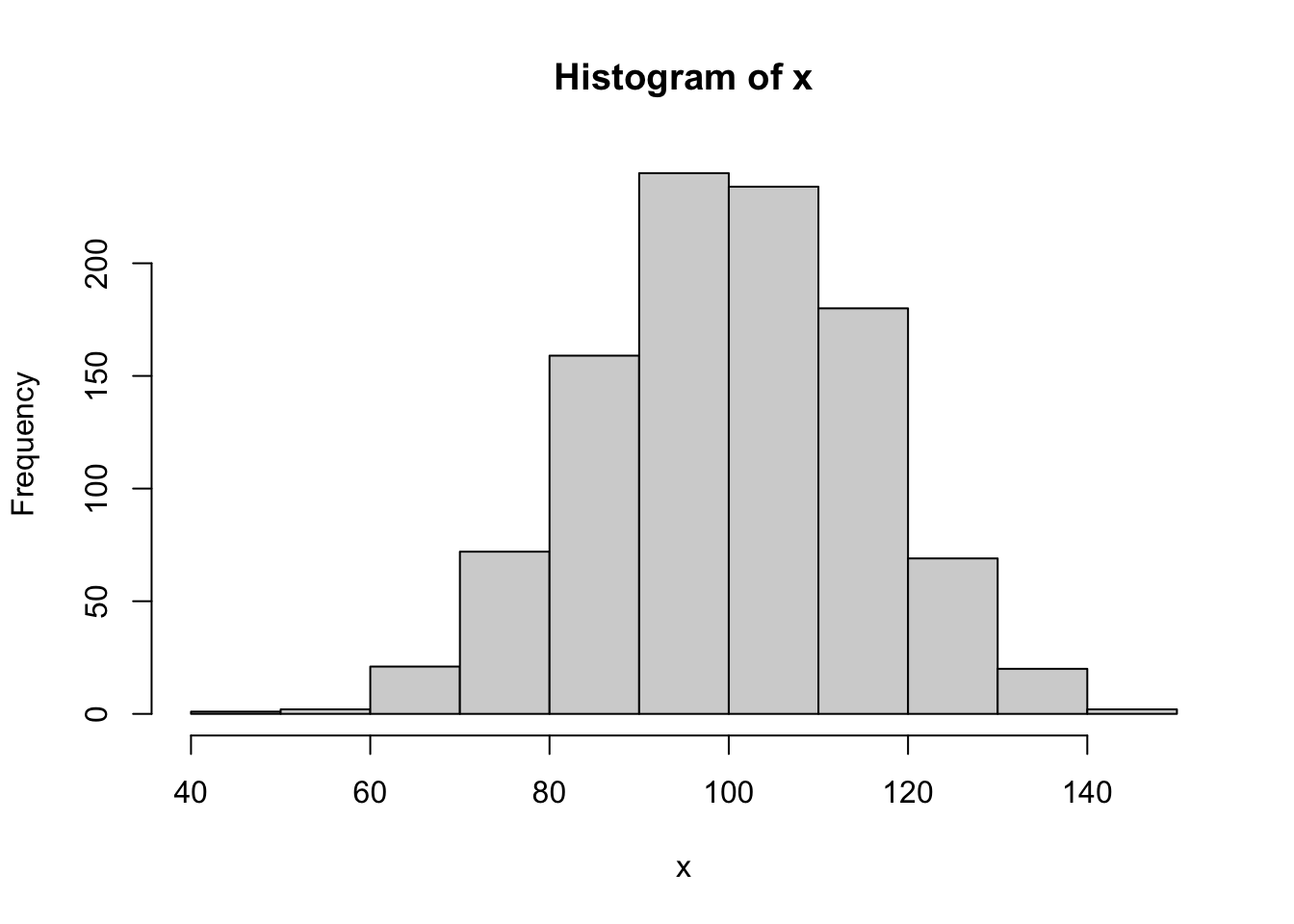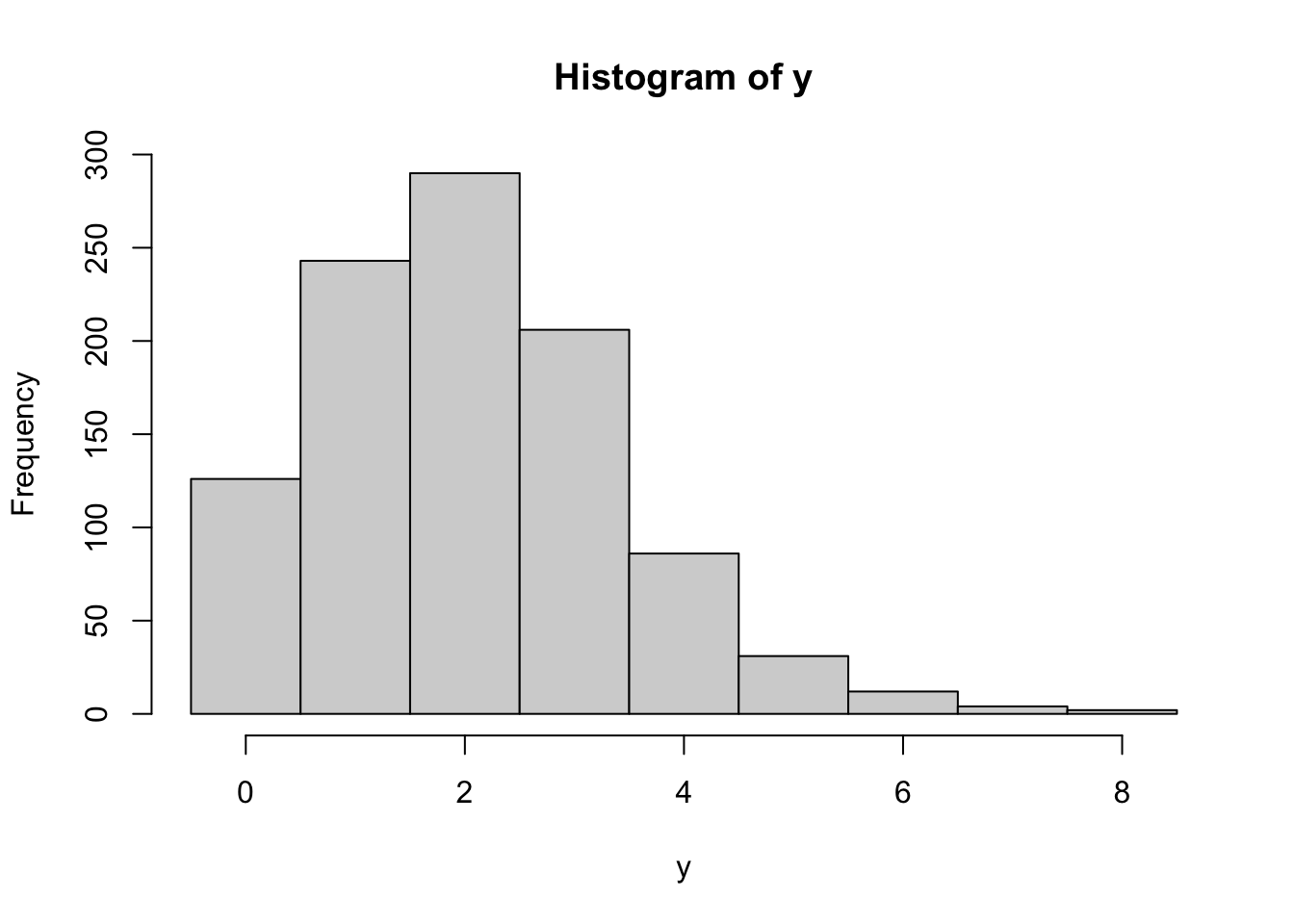The rand_bytes function binds to RAND_bytes in OpenSSL to generate cryptographically strong pseudo-random bytes. See the OpenSSL documentation for what this means.

rnd <- rand_bytes(10)
print(rnd)
  47 c3 99 90 9a fc ea 9d a5 0a

Bytes are 8 bit and hence can have 2^8 = 256 possible values.

as.numeric(rnd)
   71 195 153 144 154 252 234 157 165  10

Each random byte can be decomposed into 8 random bits (booleans)

x <- rand_bytes(1)
as.logical(rawToBits(x))
  TRUE FALSE FALSE FALSE FALSE FALSE  TRUE  TRUE

## Secure Random Numbers

rand_num is a simple (2 lines) wrapper to rand_bytes to generate random numbers (doubles) between 0 and 1.

rand_num(10)
  0.47892176 0.83259337 0.71769812 0.30193133 0.32489348 0.14622749
 0.78829348 0.27218616 0.07829514 0.30813344

To map random draws from [0,1] into a probability density, we can use a Cumulative Distribution Function. For example we can combine qnorm and rand_num to simulate rnorm:

# Secure rnorm
x <- qnorm(rand_num(1000), mean = 100, sd = 15)
hist(x)Same for discrete distributions:

# Secure rbinom
y <- qbinom(rand_num(1000), size = 20, prob = 0.1)
hist(y, breaks = -.5:(max(y)+1))